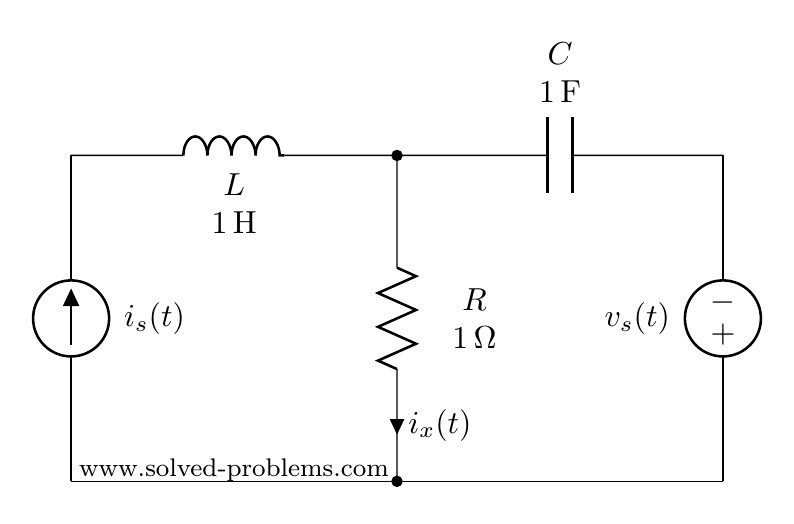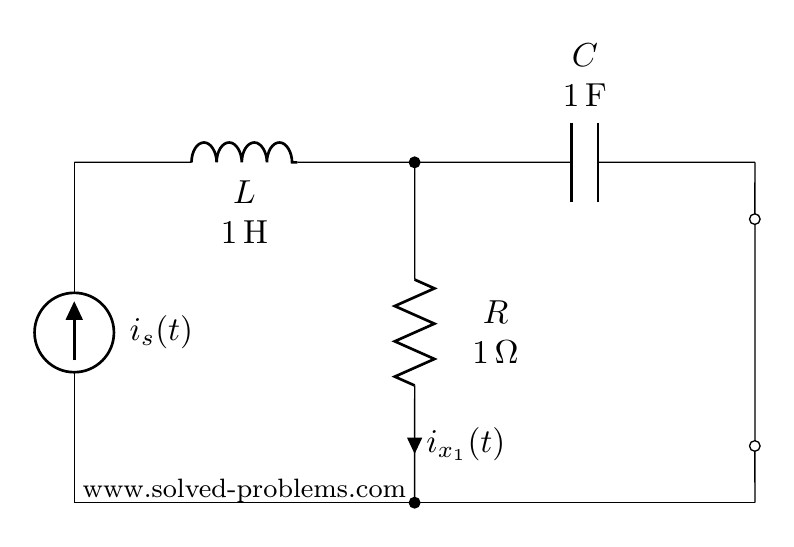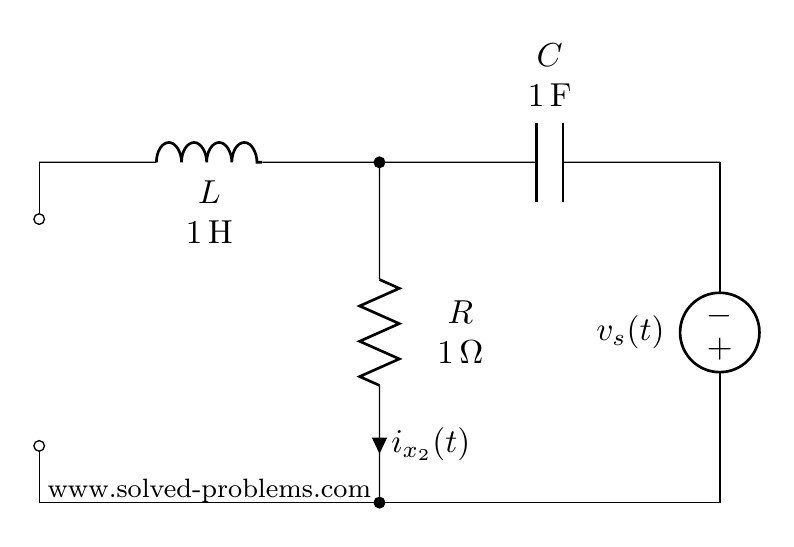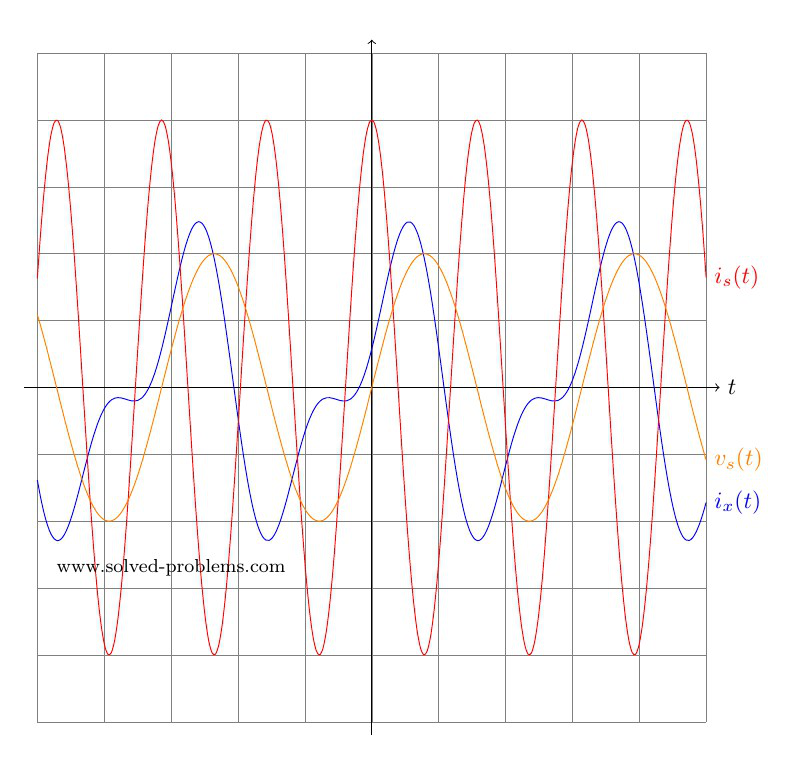In AC circuit analysis, if the circuit has sources operating at different frequencies, Superposition theorem can be used to solve the circuit. Please note that AC circuits are linear and that is why Superposition theorem is valid to solve them.

## Problem

Determinewhereand.## Solution with AC Circuit Analysis

Since sources are operating at different frequencies, i.e.and, we have to use the Superposition theorem. That is to say that we need to determine contribution of each source on. Then, the final answer is to obtained by adding the individual responses in the time domain. Please note that, since the impedances depend on frequency, we need to have a different frequency-domain circuit for each frequency.

### Contribution of the current source

To find the contribution of the current source, we need to turn off other source(s). So, we need to turn off the voltage source. This is very similar to DC circuits that we discussed before:

Voltage sources become a short circuit when turned off.#### Frequency domain

We first convert the circuit to the frequency domain:Using current divider:#### Time domain

Conversion to time-domain:Please note that the sinusoidal function foris cosine and consequently, cosine must be used in convertingto the time domain.

### Contribution of the voltage source

To find the contribution of the voltage source, the current source needs to be turned off. As mentioned before:

To turn off a current source it should be replaced by an open circuit#### Frequency domainAs the inductor branch is open, this is a very simple circuit with three elements in series:,and. Therefore,#### Time domain(why?)

Now that we have determined bothandin time domain, we can go ahead and add them up to find. Please note that we could not addandbecause they are not phasors with the same frequency.Hi! Yaz is here. I am passionate about learning and teaching. I try to explain every detail simultaneously with examples to ensure that students will remember them later too.

## Join the Conversation

1.2.3.1.Eng. Ahmad Khaled says:

Very good work.

2.Pablo says:

Very good work indeed

3.jafar says:

Should not the sin be with minus ?
I mean ix2 has an opposite direction for the calculated one